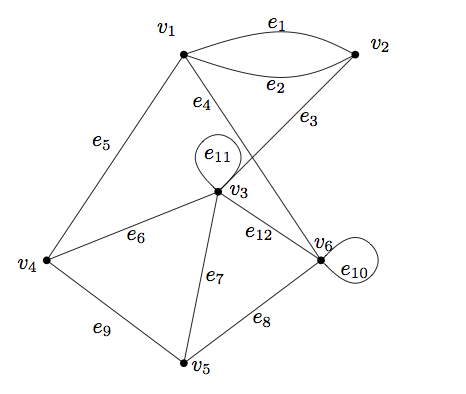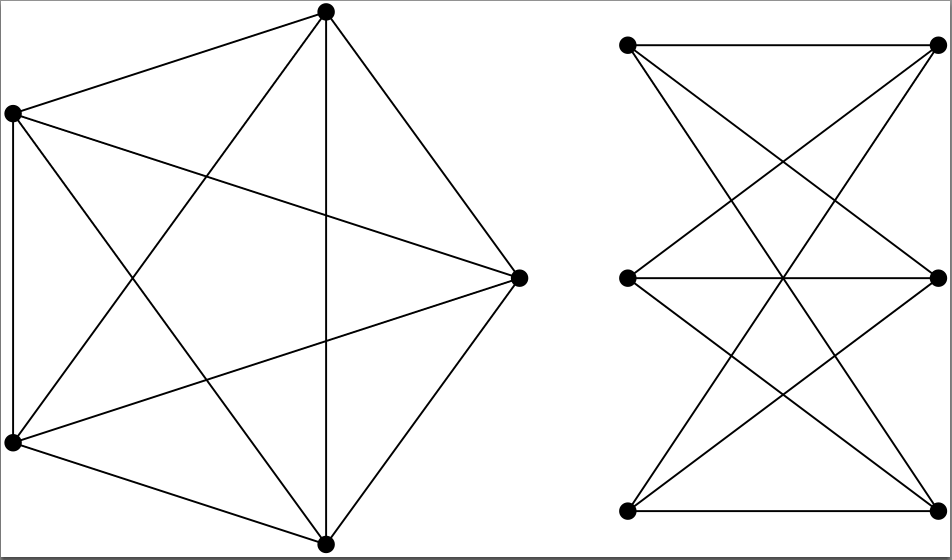# Rota’s Conjecture proved!

The Twitterverse has been abuzz recently with the news that Rota’s Conjecture has been proved by Jim Geelen, Bert Gerards, and Geoff Whittle! See press releases here and here (with an interview of Geoff at the bottom) and here. This news was known, by word of mouth, to people in the community close to the sources for a while, but let me take this opportunity to try to explain to the non-experts what this conjecture is all about. I will build up in a few steps from a small class of objects to bigger and bigger classes. For the experts, this survey by Geelen, Gerards, and Whittle might be better reading.

# 1. Graphs

Kuratowski’s Theorem (or, more correctly, a variant proved by Wagner), states:

Theorem 1. A graph is planar if and only if it has no minor isomorphic to $K_5$ or $K_{3,3}$.

A number of words need definition. A graph is a triple $(V, E, \iota)$ where $V$ is a finite set, called the vertices, $E$ is a finite set, called the edges, and $\iota: V\times E \to \{0,1\}$ is an incidence relation telling us which vertices meet which edges, with the rule that every edge meets exactly one or two vertices. Such structures are easily visualized as networks:A graph is planar if it can be drawn in the plane such that no two edges cross. The drawing above does not meet this criterion because edges $e_3$ and $e_4$ cross, but it is not hard to fix this, so the graph itself is planar.

minor of a graph is obtained by doing a sequence of the following operations: delete an edge, contract an edge (this means deleting the edge and then identifying its two endpoints), or delete a vertex having no edges incident with it. Finally, the two graphs $K_5$ and $K_{3,3}$ are the following:We say $K_{5}$ and $K_{3,3}$ are the excluded minors for the class of planar graphs. Now Robertson and Seymour proved an amazing and vast generalization of this:

Theorem 2. Every minor-closed class of graphs can be characterized by a finite set of excluded minors.

“Minor-closed” means that if a graph is in the class, then all its minors are as well. This was a work of epic proportions, spanning 23 papers and hundreds of pages.

# 2. Binary matroids

Let $G$ be a graph, and consider the following matrix: label the rows by $V$, the columns by $E$, and an entry $A_{ve}$ is 1 if $v$ is incident with $e$ in $G$, and 0 otherwise. This is called the incidence matrix of $G$. For many reasons, instead of looking at the matrix by itself, we look at its row space (i.e. every vector that can be obtained by taking linear combinations of the rows). Here we interpret the matrix over $\text{GF}(2)$, i.e. the finite field with 2 elements (so $1+1 = 0$). Just like we wanted to distinguish planar graphs among all graphs, we want to distinguish row spaces of graphs among all row spaces:

Problem. Which row spaces can be obtained as the row space of the incidence matrix of a graph?

The following classical result is due to Tutte:

Theorem 3. A row space over $\text{GF}(2)$ is the row space of a graph if and only if it does not have any minor isomorphic to one of the following row spaces: $M^*(K_5)$, $M^*(K_{3,3})$, $F_7$, $F_7^*$.

Again a finite list! Of course, I have to tell you what it means to have another row space as a minor. We now have only two operations, deletion and contraction. Deletion simply means deleting one coordinate from each vector in the row space (this corresponds to deleting a column from the original matrix). Contraction means that first we keep only the vectors that have a 0 in the specified coordinate, and then we delete that coordinate (in the matrix, we’d row-reduce the column so it has only one non-zero entry in the first row, and then we delete both that first row and the column). You can check that this corresponds to deletion and contraction in the graph.

The row space $F_7$ is given by the following matrix:

$$\begin{bmatrix} 1 & 0 & 0 & 1 & 1 & 0 & 1\\ 0 & 1 & 0 & 1 & 0 & 1 & 1\\ 0 & 0 & 1 & 0 & 1 & 1 & 1\end{bmatrix}$$

Finally, the row spaces indicated with a star are the orthogonal complements of these row spaces.

Note that Robertson and Seymour’s result does not help at all in the proof of Tutte’s theorem: the former talks only about minors inside the class of graphs, and Tutte’s theorem talks about minors just outside the class.

Binary row spaces are a much bigger set of objects than graphs (see Peter’s posts for more precise statements). Nevertheless, Geelen, Gerards, and Whittle managed to prove an analogue of the Robertson-Seymour theorem:

Theorem 4. Every minor-closed class of binary row spaces can be characterized by a finite set of excluded minors.

This (and the generalization discussed below) took them over a decade to prove, and they are currently working on a write-up, which they project will take another few years to complete.

# 3. Matroids

To define a matroid, we again look at the row space slightly differently. Take a matrix generating the row space, and let $E$ be the set of columns. We say a subset of $E$ is independent if the corresponding column vectors are linearly independent, and it is dependent otherwise. Now we do something weird: we throw away all information about the vectors, and only keep the independence information. What we’re left with is an example of a matroid.

Definition. matroid is a pair $(E, \mathcal{I})$ where $E$ is a finite set and $\mathcal{I}$ is a collection of subsets of $E$, called independent sets, that satisfy the following axioms:

1. $\emptyset \in \mathcal{I}$;
2. If $X \in \mathcal{I}$ and $Y \subseteq X$ then $Y \in \mathcal{I}$;
3. If $X, Y \in \mathcal{I}$ and $Y$ has more elements than $X$, then there exists $e\in Y – X$ such that $X\cup\{e\} \in \mathcal{I}$.

It is easily verified that these hold for the example defined in the first paragraph. But they don’t just hold for the row spaces over $\text{GF}(2)$, they hold for any matrix! This prompts new terminology: a matroid is $\mathbb{F}$-representable if there exists a matrix over the field $\mathbb{F}$ whose columns satisfy the dependencies prescribed by the matroid. Now we’ve once again grown our collection of objects, and therefore we would like to identify the old objects inside the new collection. Tutte has done this for us:

Theorem 5. A matroid is $\text{GF}(2)$-representable if and only if it has no minor isomorphic to $U_{2,4}$.

Here $U_{2,4}$ is the matroid $(E, \mathcal{I})$ where $E = \{1,2,3,4\}$ and $\mathcal{I}$ consists of all subsets of size at most 2. The minor operation again generalizes the operations above: deletion of $e$ means we restrict our matroid to $E – \{e\}$ and the independent sets to those not containing $e$. Contraction of $e$ means the same, but in addition we require keeping only those independent sets $X$ such that $X\cup \{e\}$ was independent in the original matroid.

Now, at last, we have all ingredients to formulate

Rota’s Conjecture. For every finite field $\text{GF}(q)$, there is a finite set of excluded minors for the class of $\text{GF}(q)$-representable matroids.

Besides the result for $q = 2$ mentioned above, the conjecture has been proved for $q = 3$ (in 1979) and $q = 4$ (in 2000). And now Geelen, Gerards, and Whittle have proved it, in one fell swoop, for all remaining finite fields. Note that the corresponding statement for infinite fields (say matrices over the real numbers) is false.

A major ingredient in their proof is the following result, an equivalent of Theorem 4 for any finite field:

Theorem 6. For every finite field $\text{GF}(q)$, every minor-closed class of $\text{GF}(q)$-representable matroids has a finite set of $\text{GF}(q)$-representable excluded minors.

Note that once again, this result (whose importance cannot be overstated, and whose proof is of an astounding complexity) talks only about matroids that are within the class of $\text{GF}(q)$-representable matroids; Rota’s Conjecture talks about matroids just outside that class. The major breakthrough that led to the proof of Rota’s Conjecture was an insight that allowed them to use the machinery they developed to prove Theorem 6 on a slightly broader class of matroids anyway.

I tried to keep this description short, and in doing so I have omitted many subtleties (to the point of making some false statements, in particular in the case of contraction, where I omitted degenerate cases). But the purpose was to give a flavor of Rota’s Conjecture, and I hope I succeeded in that.

For a thorough introduction to matroid theory I recommend the book by James Oxley. For a survey of Geelen, Gerards, and Whittle’s work on matroid minors, see here. The survey will also have references for all results I mentioned above. I’m sure that we will frequently return to the subject of Rota’s Conjecture in this blog, hopefully with a more technical account of the proof techniques.

1.Dan on said: